# Glossary of EM.Cube's Observables, Data & Graph Types

(diff) ← Older revision | Latest revision (diff) | Newer revision → (diff)

## 3D Cartesian Data Observable

FUNCTION: Used to import a 3D Cartesian spatial data file with a ".CAR" file extension and visualize its content overlaid on the project workspace

TO DEFINE A 3D CARTESIAN DATA:

1. Right-click on the Cartesian Data item in the navigation tree.
2. Select Insert New Observable... to open up the Cartesian Data Visualization Dialog.
3. Accept the default settings or modify them. You can select the visualization type from two options: Cube (Intensity) and Vector.
4. Click the OK button of this dialog to open up the standard Windows Open dialog. The file type is set to .CAR. Browse your folders to select the .CAR file you want to import.
5. After importing the Cartesian data file, new items are added under the observable's name in the navigation tree.

PYTHON COMMAND: cartesian_data(file_name,vis_type)

CARTESIAN DATA PARAMETERS:

Parameter Name Value Type Units Default Value Notes
max_size real numeric project units 2 orientation of field sensor plane
cone_length_ratio real numeric project units 0.5 ratio of the vector's cone height to the total arrow length
cone_radius_ratio real numeric project units 0.25 ratio of the vector's cone radius to the total arrow length

CARTESIAN DATA OUTPUT DATA FILES

Data File Name Data Type Description Notes & Restrictions
FileName.CAR 3D real or complex, scalar or vector data generic spatial data CubeCAD, EM.Tempo & EM.Ferma

## Bar Chart

ICON: None

MODULE: EM.Terrano

FUNCTION: Displays the values of a dependent variable as vertical bars of proportional heights versus a discrete set of values of an independent variable

TO PLOT A BAR CHART:

1. The delay profile of the rays received by a specified receiver can be plotted on a bar chart.
2. Open EM.Terrano's Data Manager, select a delay profile data file with name similar to "SBR_ReceiverSet_1_DELAY.DAT", and click the Plot button.

PYTHON COMMAND(S): None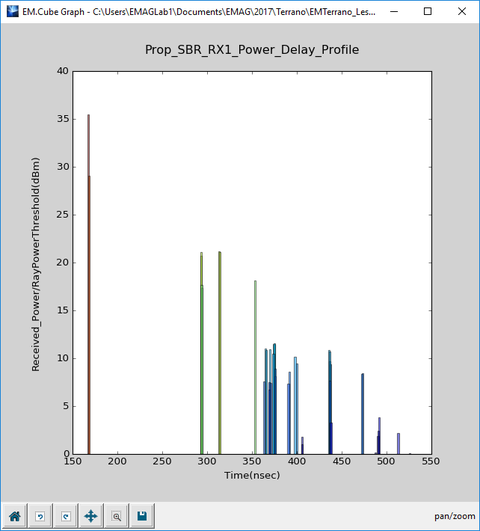A typical bar chart of a receiver's delay profile.

## Cartesian Graph

ICON: None

FUNCTION: Displays the variation of a real-valued scalar data set

TO PLOT A CARTESIAN GRAPH:

1. Select one or more data files with a .DAT file extension in the Data Manager and click the Plot button.
2. Cartesian data are plotted in linear scale by default. You can change the scale to dB-Field or dB-Power. To do so, while the data file is selected in the Data Manager, click the Graph Settings button. In the graph settings dialog, choose the one of the Linear, dB_Field or dB_Power options from the drop-down list labeled Data Format. Close the graph settings dialog and return to the data manager. Plot the selected data file once again.
3. Alternatively, you can define two NumPy vectors X and Y in the Python Interpreter and use the emag_plot_cartesian(...) function.
4. Or you can define a NumPy matrix or load it into the Python Interpreter and use the emag_plot_multi(...) function.

PYTHON COMMAND(S): emag_plot_dat(file_name,auto_scale=True,title="",xlabel="Default",ylabel="Default",FRColor=(1,1,1),BKColor=(1,1,1),PLColor=(1,0,0),linewidth=1,linestyle="solid",marker="None",margins=(0.15,0.1,0.1,0.1,0.2,0.2,0.8,0.9,0.5,0.5),show_major_grid=True,show_minor_grid=False,MAJColor=(0.25,0.25,0.25),MINColor=(0.5,0.5,0.5),major_grid_linewidth=1,minor_grid_linewidth=1,major_grid_linestyle="dotted",minor_grid_linestyle="dotted",axis_param=(0,0,5,5,0,0,5,5),show_legend=True,legend_label1="Default",legend_label2="Default",show_textbox=False,annot_text="",font_size=10,TXColor=(0,0,0),*args,**kwargs)

emag_plot_two_dat(file_name1,file_name2,auto_scale=True,title="",xlabel="Default",ylabel="Default",FRColor=(1,1,1),BKColor=(1,1,1),PLColor1=(1,0,0),linewidth1=1,linestyle1="solid",marker1="None",PLColor2=(1,0,0),linewidth2=1,linestyle2="solid",marker2="None",margins=(0.15,0.1,0.1,0.1,0.2,0.2,0.8,0.9,0.5,0.5),show_major_grid=True,show_minor_grid=False,MAJColor=(0.25,0.25,0.25),MINColor=(0.5,0.5,0.5),major_grid_linewidth=1,minor_grid_linewidth=1,major_grid_linestyle="dotted",minor_grid_linestyle="dotted",axis_param=(0,0,5,5,0,0,5,5),show_legend=True,legend_label1="Default",legend_label2="Default",show_textbox=False,annot_text="",font_size=10,TXColor=(0,0,0),*args,**kwargs)

emag_plot_cartesian(x,y,title="New Graph",x_label="x",y_label="y",*args,**kwargs)

emag_plot_multi(a_matrix,x_col,y_col_list,title="New Graph",x_label="x",y_label="cols",*args,**kwargs)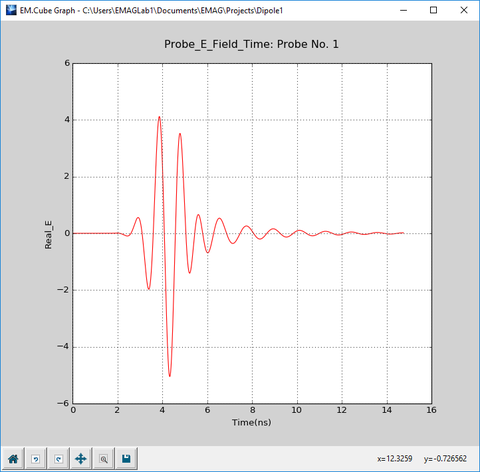A typical Cartesian graph of a temporal waveform.A typical Cartesian graph of electric field distributions on two sensor planes along the X-axis.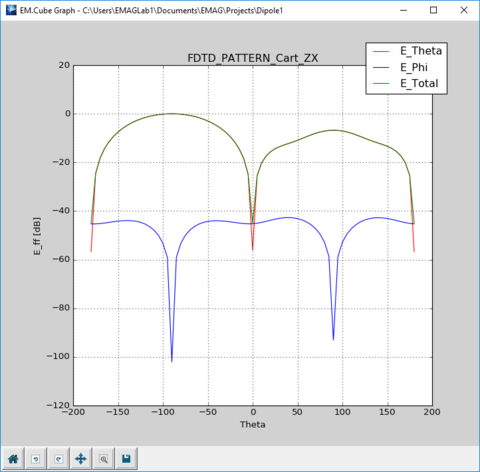A typical Cartesian graph of 2D far-field radiation pattern in a principal plane.

## Colorgrid Plot

ICON: None

FUNCTION: Displays the color-coded intensity plot of a Cartesian spatial data set of the form z = f(x,y) on a specified principal plane

TO PLOT A CARTESIAN GRAPH:

1. You can visualize 3D Cartesian data, receiver power coverage maps or electric and magnetic field distributions as colorgrid plots.
2. Select a 3D Cartesian data file with a .CAR file extension or a coverage data file with a .COV file extension or a field sensor data file with a .SEN file extension in the Data Manager's "3D Data Files" tab and click the Graph Settings button.
3. From the drop-down list labeled Graph Type, choose the Surface option. Close the graph settings dialog and return to the Data Manager. With the 3D data file selected, click the Plot button of Data Manager.
4. Alternatively, you can define two NumPy vectors X and Y and a matrix Z in the Python Interpreter and use the emag_plot_surface(...) function.

PYTHON COMMAND(S): emag_plot_colorgrid(x,y,z_matrix,title="New Graph",x_label="x",y_label="y",*args,**kwargs)

emag_plot_car_grid(file_name,title="",FRColor=(1,1,1),BKColor=(1,1,1),margins=(0.15,0.1,0.1,0.1,0.2,0.2,0.8,0.9,0.5,0.5),*args,**kwargs)

emag_plot_cov(file_name,title="",FRColor=(1,1,1),BKColor=(1,1,1),margins=(0.15,0.1,0.1,0.1,0.2,0.2,0.8,0.9,0.5,0.5),*args,**kwargs)

emag_plot_sen_grid(file_name,title="",FRColor=(1,1,1),BKColor=(1,1,1),margins=(0.15,0.1,0.1,0.1,0.2,0.2,0.8,0.9,0.5,0.5),*args,**kwargs)

emag_plot_sen_tempo_grid(file_name,input_name,grid_name,title="",FRColor=(1,1,1),BKColor=(1,1,1),margins=(0.15,0.1,0.1,0.1,0.2,0.2,0.8,0.9,0.5,0.5),*args,**kwargs)

emag_plot_sen_ferma_grid(file_name,title="",FRColor=(1,1,1),BKColor=(1,1,1),margins=(0.15,0.1,0.1,0.1,0.2,0.2,0.8,0.9,0.5,0.5),*args,**kwargs)A typical colorgrid plot of a coverage map.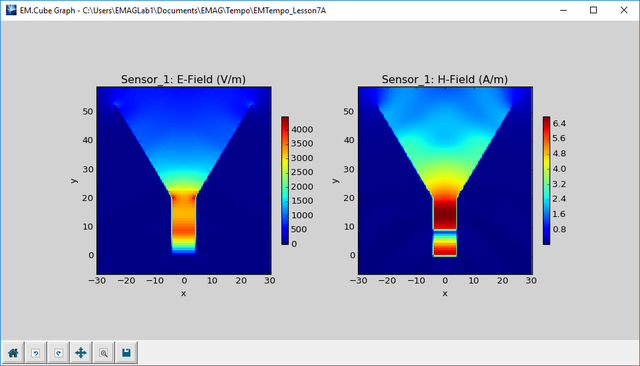A typical colorgrid plot of the electric and magnetic field distributions.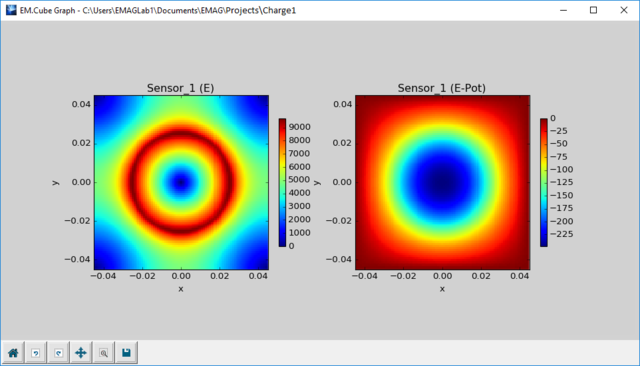A typical colorgrid plot of the electric field and electric potential.

## Combined Multi-Column Plot

ICON: None

FUNCTION: Displays the variation of several real-valued scalar data sets with a common independent variable as a family of Cartesian curves. The individual curves represent the variation of dependent variable as a function of the last independent variable for fixed combinations of the other independent variables.

TO PLOT A CARTESIAN GRAPH:

1. Select a data file with a .DAT file extension in the Data Manager and click the Graph Settings button.
2. In the Graph Settings dialog, select the Multivariable Datasets option from the Graph Type drop-down list. Click the OK button to close the dialog and return to the Data Manager.
3. Click the Plot button of Data Manager to plot the combined multi-column graph.

PYTHON COMMAND(S): emag_plot_multi_dat(file_name,auto_scale=True,title="",xlabel="Default",ylabel="Default",FRColor=(1,1,1),BKColor=(1,1,1),PLColor1=(1,0,0),linewidth1=1,linestyle1="solid",marker1="None",PLColor2=(0,0,1),linewidth2=1,linestyle2="solid",marker2="None",PLColor3=(0,1,0),linewidth3=1,linestyle3="solid",marker3="None",margins=(0.15,0.1,0.1,0.1,0.2,0.2,0.8,0.9,0.5,0.5),show_major_grid=True,show_minor_grid=False,MAJColor=(0.25,0.25,0.25),MINColor=(0.5,0.5,0.5),major_grid_linewidth=1,minor_grid_linewidth=1,major_grid_linestyle="dotted",minor_grid_linestyle="dotted",axis_param=(0,0,5,5,0,0,5,5),show_legend=True,legend_label1="Default",legend_label2="Default",legend_label3="Default",show_textbox=False,annot_text="",font_size=10,TXColor=(0,0,0),*args,**kwargs)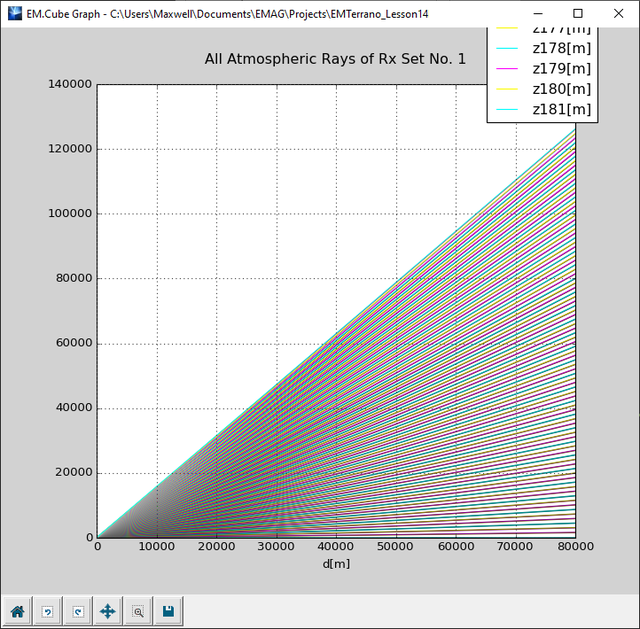A typical combined multi-column plot of the launched rays at a ray angular resolution of 1°.

## Complex Data Graph

ICON: None

MODULE: EM.Tempo, EM.Picasso, EM.Libera

FUNCTION: Displays the variation of a complex-valued scalar data set

TO PLOT A COMPLEX DATA GRAPH:

1. Select one or more data files with a .CPX file extension in the Data Manager and click the Plot button.
2. Alternatively, you can define a real-valued NumPy vector X and a complex-valued NumbPy vector Z in the Python Interpreter and use the emag_plot_cartesian(...) function.
3. Complex data graph can be plotted in two different formats: "Mag + Phase" or "Real + Imag". The former plots the magnitude and phase of the complex-valued dependent variable on two separate graphs. The latter plots the

real and imaginary parts of the complex-valued dependent variable on same graph.

PYTHON COMMAND(S): emag_plot_cpx(file_name,auto_scale=True,title="",xlabel="Default",ylabel="Default",FRColor=(1,1,1),BKColor=(1,1,1),PLColor1=(1,0,0),linewidth1=1,linestyle1="solid",marker1="None",PLColor2=(1,0,0),linewidth2=1,linestyle2="solid",marker2="None",margins=(0.15,0.1,0.1,0.1,0.2,0.2,0.8,0.9,0.5,0.5),show_major_grid=True,show_minor_grid=False,MAJColor=(0.25,0.25,0.25),MINColor=(0.5,0.5,0.5),major_grid_linewidth=1,minor_grid_linewidth=1,major_grid_linestyle="dotted",minor_grid_linestyle="dotted",axis_param=(0,0,5,5,0,0,5,5),show_legend=True,legend_label1="Default",legend_label2="Default",show_textbox=False,annot_text="",font_size=10,TXColor=(0,0,0),*args,**kwargs)

emag_plot_two_cpx(file_name1,file_name2,auto_scale=True,title="",xlabel="Default",ylabel="Default",FRColor=(1,1,1),BKColor=(1,1,1),PLColor1=(1,0,0),linewidth1=1,linestyle1="solid",marker1="None",PLColor2=(1,0,0),linewidth2=1,linestyle2="solid",marker2="None",margins=(0.15,0.1,0.1,0.1,0.2,0.2,0.8,0.9,0.5,0.5),show_major_grid=True,show_minor_grid=False,MAJColor=(0.25,0.25,0.25),MINColor=(0.5,0.5,0.5),major_grid_linewidth=1,minor_grid_linewidth=1,major_grid_linestyle="dotted",minor_grid_linestyle="dotted",axis_param=(0,0,5,5,0,0,5,5),show_legend=True,legend_label1="Default",legend_label2="Default",show_textbox=False,annot_text="",font_size=10,TXColor=(0,0,0),*args,**kwargs)

emag_plot_complex(x,z_complex,plot_type="mag+phase",title="New Graph",x_label="x",*args,**kwargs)A typical complex data graph with the "Mag + Phase" option.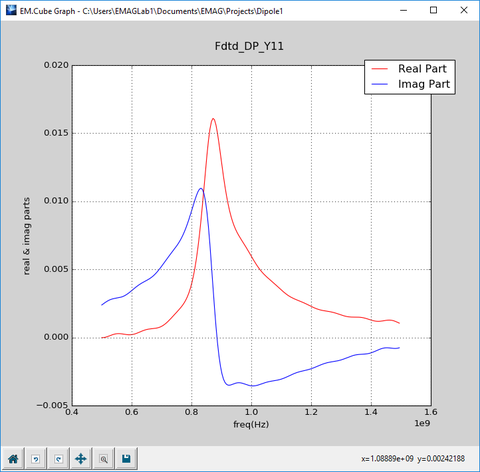A typical complex data graph with the "Real + Imag" option.

## Contour Plot

ICON: None

FUNCTION: Displays the contour plot of a Cartesian spatial data set of the form z = f(x,y) on a specified principal plane

TO PLOT A CARTESIAN GRAPH:

1. You can visualize 3D Cartesian data, receiver power coverage maps or electric and magnetic field distributions as contour plots.
2. EM.Cube offers two types of contour plots: regular contour plots and filled contour plots.
3. Select a 3D Cartesian data file with a .CAR file extension or a coverage data file with a .COV file extension or a field sensor data file with a .SEN file extension in the Data Manager's "3D Data Files" tab and click the Graph Settings button.
4. From the drop-down list labeled Graph Type, choose either of the Contour or Filled Contour options. Close the graph settings dialog and return to the Data Manager. With the 3D data file selected, click the Plot button of Data Manager.
5. Alternatively, you can define two NumPy vectors X and Y and a matrix Z in the Python Interpreter and use the emag_plot_surface(...) function.

PYTHON COMMAND(S): emag_plot_contour(x,y,z_matrix,filled=False,title="New Graph",x_label="x",y_label="y",*args,**kwargs)

emag_plot_car_contour(file_name,filled=False,title="",FRColor=(1,1,1),BKColor=(1,1,1),margins=(0.15,0.1,0.1,0.1,0.2,0.2,0.8,0.9,0.5,0.5),*args,**kwargs)

emag_plot_cov_contour(file_name,filled=False,title="",FRColor=(1,1,1),BKColor=(1,1,1),margins=(0.15,0.1,0.1,0.1,0.2,0.2,0.8,0.9,0.5,0.5),*args,**kwargs)

emag_plot_sen_contour(file_name,filled=False,title="",FRColor=(1,1,1),BKColor=(1,1,1),margins=(0.15,0.1,0.1,0.1,0.2,0.2,0.8,0.9,0.5,0.5),*args,**kwargs)

emag_plot_sen_tempo_contour(file_name,input_name,grid_name,filled=False,title="",FRColor=(1,1,1),BKColor=(1,1,1),margins=(0.15,0.1,0.1,0.1,0.2,0.2,0.8,0.9,0.5,0.5),*args,**kwargs)

emag_plot_sen_ferma_contour(file_name,filled=False,title="",FRColor=(1,1,1),BKColor=(1,1,1),margins=(0.15,0.1,0.1,0.1,0.2,0.2,0.8,0.9,0.5,0.5),*args,**kwargs)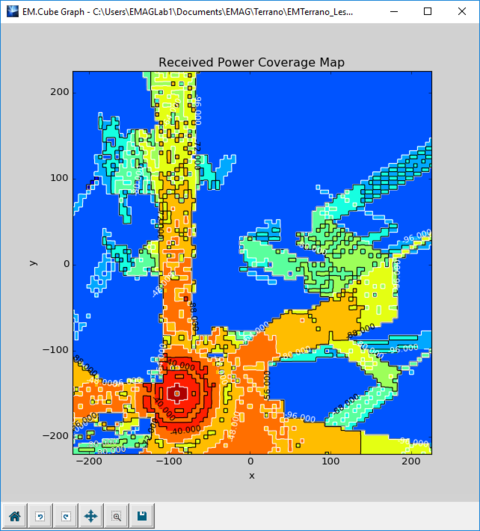A typical filled contour plot of a coverage map.A typical contour plot of electric field and potential.A typical filled contour plot of electric field and potential.

## Current Distribution Observable

MODULE: EM.Illumina, EM.Picasso, EM.Libera

FUNCTION: Computes either frequency-domain electric and magnetic current components on the mesh

TO DEFINE A CURRENT DISTRIBUTION:

1. Right-click on the Current Distributions item in the navigation tree.
2. Select Insert New Observable... to open up the Current Distribution Dialog.
3. This observable doesn't have any parameters to set.
4. Click the OK button of the dialog to return to the project workspace.

In EM.Picasso, you need to define a current distribution observable for each individual trace or embedded object set. You select the desired trace from a drop-down list. In EM.Libera and EM.Illumina, a single current distribution observable is defined for the entire physical structure.

PYTHON COMMAND: current_dist(label)

CURRENT DISTRIBUTION PARAMETERS: None

CURRENT DISTRIBUTION'S OUTPUT DATA FILES

Data File Name Data Type Description Notes & Restrictions
ObservableName.CUR 3D complex vector data frequency-domain Jx, Jy, Jz, Mx, My, Mz current data EM.Illumina & EM.Libera
TraceName.CUR 3D complex vector data frequency-domain Jx, Jy, Jz, Mx, My, Mz current data EM.PicassoEM.Picasso's current distribution dialog.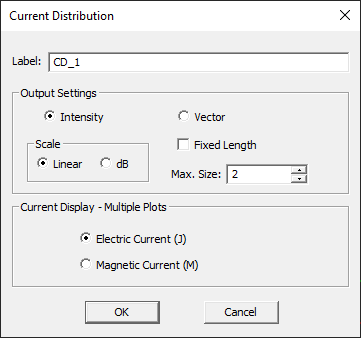EM.Illumina's current distribution dialog.

## Custom Output Parameters

You can use the "standard output parameters" to define new "custom output parameters". In many cases, the standard output may not be what you are looking for. For example, the directivity D0 of a radiating structure is stored in linear scale. If you are rather interested in dB-scaled directivity values, you can define a new output parameter for that purpose and store the results in an ASCII data file. Custom output parameters are written into ASCII data files that bear the name of the parameter with a .DAT file extension. At the end of a simulation, you can see a list of all the custom output data files in Data Manager. Just like other standard output, you can plot these files or perform data operations on them.

To define a new custom output parameter, follow the procedure below:

• Click the Custom Outputbutton of the Simulate Toolbar or select menu → Simulate → Custom Output or use the keyboard shortcut Ctrl+K. This opens up the Custom Output dialog, which is initially empty. You need to define your new custom output parameters one by one and add them to this list.
• To define a new parameters, click the Add button of the dialog.
• A new dialog opens up, which offers a list of all the available standard output parameters for your information. You need to choose a name or Label for your custom output and enter an Expression for its definition. This can be any mathematical expression that involves the standard output parameters, arithmetic operations, and one ore more EM.Cube's functions. Double-clicking on the name of a standard output parameter in the dialog's table will copy its name to the expression box.
• Once you are done with the definition of your new custom output parameter, click the OK button to add it to the Custom Output list.

The button labeled f(x) at the bottom of this dialog can be used to open EM.Cube's Function List to review the format or syntax of these functions.Defining a new custom output parameter.

## EM.Grid

ICON: None

KEYBOARD SHORTCUT: Ctrl+Shift+G

FUNCTION: Opens EM.Grid for plotting data files

PYTHON COMMAND: plot_file(filename)EM.Grid showing a 2D radiation pattern plot.

## Energy-Power Observable

MODULE: EM.Tempo

FUNCTION: Instructs EM.Tempo to compute the energy and power quantities for the entire computational domain in the time domain as well as energy and power density quantities on all field sensor planes in the frequency domain

TO DEFINE A ENERGY-POWER OBSERVABLE:

1. Right-click on the Domain Energy item in the navigation tree of EM.Tempo.
2. Select Compute Domain Energy from the contextual menu.
3. A check mark is placed before the energy item which sets the observable.

PYTHON COMMAND: set_energy_power(enable)

ENERGY OUTPUT DATA FILES

Data File Name Data Type Description Notes & Restrictions
Domain_Energy_E.DAT 2D real scalar data the electric energy of the FDTD domain as a function of time none
Domain_Energy_H.DAT 2D real scalar data the magnetic energy of the FDTD domain as a function of time none
Domain_Total_Energy.DAT 2D real scalar data the total energy of the FDTD domain as a function of time none
Domain_Dissipated_Power.DAT 2D real scalar data the total energy of the FDTD domain as a function of time none
SensorName_Electric_Energy_Density.CAR 3D Cartesian real scalar data the electric energy density on SensorName plane none
SensorName_Magnetic_Energy_Density.CAR 3D Cartesian real scalar data the magnetic energy density on SensorName plane none
SensorName_Total_Energy_Density.CAR 3D Cartesian real scalar data the total energy density on SensorName plane none
SensorName_Dissipated_Power_Density.CAR 3D Cartesian real scalar data the dissipated power density on SensorName plane none
SensorName_SAR_Density.CAR 3D Cartesian real scalar data the specific absorption rate density on SensorName plane none
SensorName_Poynting.CAR 3D Cartesian complex vector data the complex Poynting vector on SensorName plane none

FUNCTION: Computes the far-zone electric fields in the spherical coordinate system

TO DEFINE A FAR-FIELD RADIATION PATTERN:

1. Right-click on the Far-Field Radiation Patterns item in the navigation tree.
2. Select Insert New Radiation Pattern... to open up the Radiation Pattern Dialog.
3. By default, the field probe is place at the origin of coordinates. Enter new (X,Y,Z) coordinates for the desired location.
4. Click the OK button of the dialog to return to the project workspace.

NOTES, SPECIAL CASES OR EXCEPTIONS: The far-zone fields are the asymptotic form of the near-zone fields when r → ∞ or k0r >> 1. Under this assumption, the fields propagate outward as transverse electromagnetic (TEM) waves:

$\mathbf{H^{ff}(r)} = \frac{1}{\eta_0} \mathbf{ \hat{k} \times E^{ff}(r)}$

Far fields are typically computed in the spherical coordinate system as functions of the elevation and azimuth observation angles θ and φ. Only the far-zone electric fields are typically considered as the far-zone magnetic fields are orthogonal and proportional to the electric fields. When your physical structure is excited using one of the many types of lumped sources, distributed sources, gap sources and their variations or using a short dipole source, the far fields represent the radiation pattern of your source(s) in the far zone. When your physical structure is illuminated by a plane wave source or a Gaussian beam source, the far fields represent the scattered fields or scattering patterns. In the case of a plane source, the radar cross section (RCS) of your structure is often more impotent than its radiation/scattering pattern.

PYTHON COMMAND: farfield(label,theta_incr,phi_incr)

Parameter Name Value Type Units Default Value Notes
phi angle increment real numeric degrees 5 resolution of computed radiation pattern along phi
theta angle increment real numeric degrees 5 resolution of computed radiation pattern along theta
non-principal phi plane real numeric degrees 45 additional plane cut
custom azimuth real numeric degrees 0 phi angle for computation of additional radiation characteristics
custom elevation real numeric degrees 0 theta angle for computation of additional radiation characteristics
Nx integer numeric - 1 array factor element count along X
Ny integer numeric - 1 array factor element count along Y
Nz integer numeric - 1 array factor element count along Z
spacing_x real numeric project units 0 array factor element spacing along X
spacing_y real numeric project units 0 array factor element spacing along Y
spacing_z real numeric project units 0 array factor element spacing along Z

FAR FIELD OUTPUT DATA FILES

Data File Name Data Type Description Notes & Restrictions
FarFieldName.RAD spherical complex data frequency-domain Eθ and Eφ field data none
FarFieldName_PATTERN_Cart_XY.DAT 2D real scalar data frequency-domain 2D Cartesian radiation pattern in the XY plane none
FarFieldName_PATTERN_Cart_YZ.DAT 2D real scalar data frequency-domain 2D Cartesian radiation pattern in the YZ plane none
FarFieldName_PATTERN_Cart_ZX.DAT 2D real scalar data frequency-domain 2D Cartesian radiation pattern in the ZX plane none
FarFieldName_PATTERN_Cart_Custom.DAT 2D real scalar data frequency-domain 2D Cartesian radiation pattern in the custom phi-plane custom phi is 45° by default
FarFieldName_PATTERN_Polar_XY.ANG 2D real angular data frequency-domain 2D Polar radiation pattern in the XY plane none
FarFieldName_PATTERN_Polar_YZ.ANG 2D real angular data frequency-domain 2D Polar radiation pattern in the YZ plane none
FarFieldName_PATTERN_Polar_ZX.ANG 2D real angular data frequency-domain 2D Polar radiation pattern in the ZX plane none
FarFieldName_PATTERN_Polar_Custom.ANG 2D real angular data frequency-domain 2D Polar radiation pattern in the custom phi-plane custom phi is 45° by default
Axial_Ratio.SPH spherical complex data axial ratio as a function of θ and φ axial ratio must be checked
EL.SPH spherical complex data LCP field components EL as a function of θ and φ axial ratio must be checked
ER.SPH spherical complex data RCP field components ER as a function of θ and φ axial ratio must be checked
Axial_Ratio_XY.DAT 2D real scalar data axial ratio and LCP & RCP field components (EL, ER) in the XY plane axial ratio must be checked
Axial_Ratio_YZ.DAT 2D real scalar data axial ratio and LCP & RCP field components (EL, ER) in the YZ plane axial ratio must be checked
Axial_Ratio_ZX.DAT 2D real scalar data axial ratio and LCP & RCP field components (EL, ER) in the ZX plane axial ratio must be checked
Axial_Ratio_Custom.DAT 2D real scalar data axial ratio and LCP & RCP field components (EL, ER) in the custom Phi-plane custom phi is 45° by default
DG.SPH spherical complex data directive gain as a function of θ and φ DG must be checked
DG_XY.DAT 2D real scalar data directive gain in the XY plane DG must be checked
DG_YZ.DAT 2D real scalar data directive gain in the YZ plane DG must be checked
DG_ZX.DAT 2D real scalar data directive gain in the ZX plane DG must be checked
DG_Custom.DAT 2D real scalar data directive gain in the custom Phi-plane custom phi is 45° by default
HPBW_XY.DAT 2D real scalar data half-power beam width in the XY plane HPBW must be checked
HPBW_YZ.DAT 2D real scalar data half-power beam width in the YZ plane HPBW must be checked
HPBW_ZX.DAT 2D real scalar data half-power beam width in the ZX plane HPBW must be checked
HPBW_Custom.DAT 2D real scalar data half-power beam width in the custom Phi-plane custom phi is 45° by default
MAX_SLL_XY.DAT 2D real scalar data maximum side lobe level in the XY plane MAX SLL must be checked
MAX_SLL_YZ.DAT 2D real scalar data maximum side lobe level in the YZ plane MAX SLL must be checked
MAX_SLL_ZX.DAT 2D real scalar data maximum side lobe level in the ZX plane MAX SLL must be checked
MAX_SLL_Custom.DAT 2D real scalar data maximum side lobe level in the custom Phi-plane custom phi is 45° by default
FNL_XY.DAT 2D real scalar data first null level in the XY plane first null parameters must be checked
FNL_YZ.DAT 2D real scalar data first null level in the YZ plane first null parameters must be checked
FNL_ZX.DAT 2D real scalar data first null level in the ZX plane first null parameters must be checked
FNL_Custom.DAT 2D real scalar data first null level in the custom Phi-plane custom phi is 45° by default
FNB_XY.DAT 2D real scalar data first null beam width in the XY plane first null parameters must be checked
FNB_YZ.DAT 2D real scalar data first null beam width in the YZ plane first null parameters must be checked
FNB_ZX.DAT 2D real scalar data first null beam width in the ZX plane first null parameters must be checked
FNB_Custom.DAT 2D real scalar data first null beam width in the custom Phi-plane custom phi is 45° by default
D0.DAT 2D real scalar data directivity none
MainBeam.DAT 2D real scalar data the elevation and azimuth angles of the main beam none
ARU.DAT 2D real scalar data axial ratio along the user defined azimuth and elevation direction axial ratio must be checked
DGU.DAT 2D real scalar data directive gain along the user defined azimuth and elevation direction DG must be checked
FBR.DAT 2D real scalar data front-to-back ratio measured as |Etot(θ = 0°)| / |Etot(θ = 180°)| FBR must be checked
REFF.DAT 2D real scalar data radiation efficiency requires a port definition
G0.DAT 2D real scalar data antenna gain requires a port definition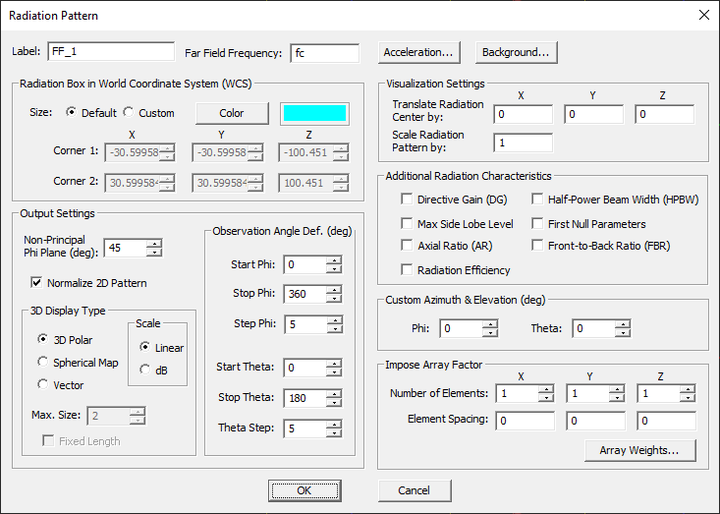EM.Tempo's far-field radiation pattern dialog.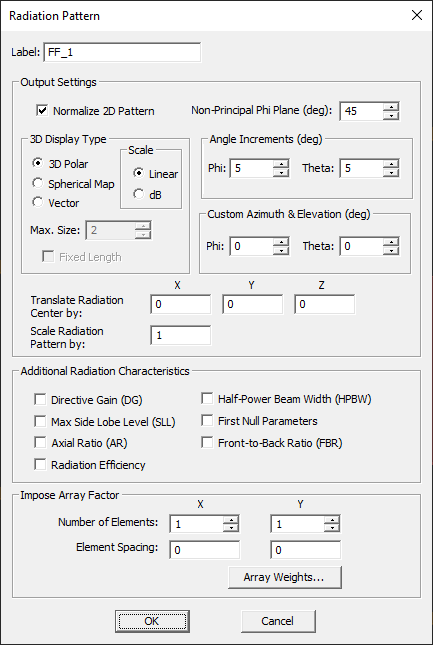EM.Picasso's far-field radiation pattern dialog.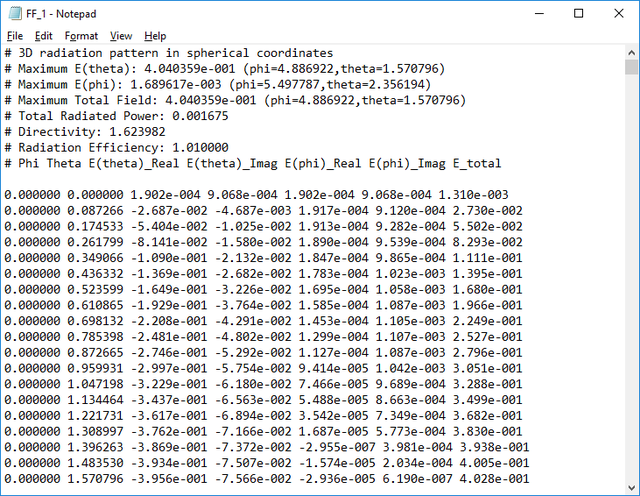Contents of a typical radiation pattern data file.

## Huygens Surface Observable

FUNCTION: Computes the tangential components of equivalent electric and magnetic surface currents on the surface of a specified Huygens box

TO DEFINE A HUYGENS SURFACE:

1. Right-click on the Huygens Surfaces item in the navigation tree.
2. Select Insert New Observable... to open up the Huygens Surface Dialog.
3. By default, the field probe is place at the origin of coordinates with a Z-orientation. Select a new orientation or enter (X,Y,Z) coordinates for the desired location.
4. Click the OK button of the dialog to return to the project workspace.

PYTHON COMMAND:

huygens_surface(label,x1,y1,z1,x2,y2,z2,xSamples,ySamples,zSamples)

huygens_surface_grid(label,x1,y1,z1,x2,y2,z2)

HUYGENS SURFACE PARAMETERS

Parameter Name Value Type Units Default Value Notes
x1 real numeric project units 0 X-coordinate of the lower front left corner of Huygens box
y1 real numeric project units 0 Y-coordinate of the lower front left corner of Huygens box
z1 real numeric project units 0 Z-coordinate of the lower front left corner of Huygens box
x2 real numeric project units 0 X-coordinate of the upper back right corner of Huygens box
y2 real numeric project units 0 Y-coordinate of the upper back right corner of Huygens box
z2 real numeric project units 0 Z-coordinate of the upper back right corner of Huygens box
Nx integer numeric - 10 sample count along X
Ny integer numeric - 1 sample count along Y
Nz integer numeric - 1 sample count along Z
near-field frequency real numeric Hz fc frequency of computation of near-field data

HUYGENS SURFACE'S OUTPUT DATA FILES

Data File Name Data Type Description
ObservableName.HUY 3D complex vector data frequency-domain Jx, Jy, Jz, Mx, My, Mz current data on the surface of the Huygens box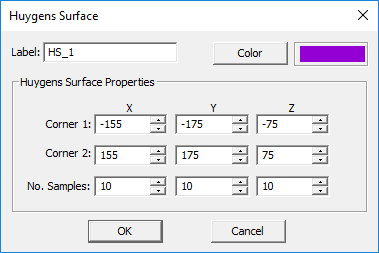EM.Libera's Huygens surface dialog.

## Multivariable Datasets Plot

ICON: None

FUNCTION: Displays the variation of a real-valued scalar data set with one, two, three or four independent variables as a family of Cartesian curves. The individual curves represent the variation of dependent variable as a function of the last independent variable for fixed combinations of the other independent variables.

TO PLOT A CARTESIAN GRAPH:

1. Select a data file with a .DAT file extension in the Data Manager and click the Graph Settings button.
2. In the Graph Settings dialog, select the Multivariable Datasets option from the Graph Type drop-down list. Click the OK button to close the dialog and return to the Data Manager.
3. Click the Plot button of Data Manager to plot the multivariable datasets graph.

PYTHON COMMAND(S): emag_plot_dat_sets(file_name,auto_scale=True,title="",xlabel="Default",ylabel="Default",FRColor=(1,1,1),BKColor=(1,1,1),PLColor1=(1,0,0),linewidth1=1,linestyle1="solid",marker1="None",PLColor2=(0,0,1),linewidth2=1,linestyle2="solid",marker2="None",PLColor3=(0,0.5,0),linewidth3=1,linestyle3="solid",marker3="None",margins=(0.15,0.1,0.1,0.1,0.2,0.2,0.8,0.9,0.5,0.5),xscale=0,yscale=0,show_major_grid=True,show_minor_grid=False,MAJColor=(0.25,0.25,0.25),MINColor=(0.5,0.5,0.5),major_grid_linewidth=1,minor_grid_linewidth=1,major_grid_linestyle="dotted",minor_grid_linestyle="dotted",axis_param=(0,0,5,5,0,0,5,5),show_legend=True,legend_label1="Default",legend_label2="Default",legend_label3="Default",show_plot1=True,show_plot2=True,show_plot3=True,show_textbox=False,annot_text="",font_size=10,TXColor=(0,0,0),*args,**kwargs)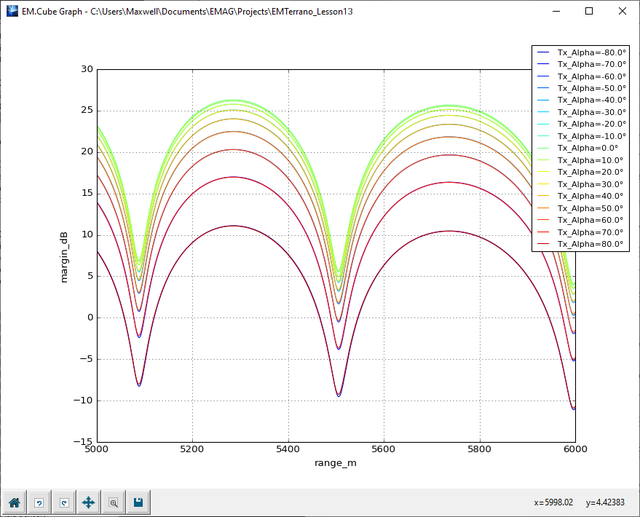A multiple dataset plot of link margin vs. both range and transmit antenna’s rotation angle.

## Near-Field Sensor Observable

FUNCTION: Computes either time-domain or frequency-domain electric and magnetic field components on a principal plane

TO DEFINE A FIELD SENSOR:

1. Right-click on the Field Sensors item in the navigation tree.
2. Select Insert New Observable... to open up the Field Sensor Dialog.
3. By default, the field sensor plane is horizontal (having a Z-orientation) and is placed at the origin of coordinates.
4. Change the orientation of the sensor plane, if necessary, or enter the (x,y,z) coordinates for a new location.
5. In EM.Tempo and EM.Ferma, the field sensor plane extends to the entire cross section of the computational domain and its resolution is driven by the mesh density.
6. In EM.Terrano, EM.Illumina, EM.Picasso or EM.Libera, you have to additionally specify the dimensions of the sensor plane as well as the number of samples along the two principal directions of the sensor plane.
7. The center coordinate that is identical to the sensor's direction determines the position of the sensor plane in the 3D space. The other two coordinates can be used to set the crosshairs of the sensor plane. These are used to compute the 2D field variations along the specified lines.
8. For the 3D visualization of the field sensor data, you have to choose one of the two plot type options: Intensity or Vector. In the case of an intensity plot (which is the default choice), you can additionally apply a data interpolation scheme to smooth out the plot. In the case of a vector plot, you can control the maximum size of the vector arrows as well as the cone ratio (ratio of the size of the conical tip to the arrow's stem).
9. If the field sensor is to be used in a sweep simulation, only the total E-field or total H-field will be recorded at each instance of the simulation. The default choice is the E-field.
10. Click the OK button of the dialog to return to the project workspace.

NOTES, SPECIAL CASES OR EXCEPTIONS: Field sensors are primarily used for the 3D visualization of electric and magnetic field distributions either in the frequency or time domain. In EM.Tempo, you can use a field sensor to record the evolution of the fields as a function of time. You can also record the field sensor data during a sweep simulation (either a frequency sweep or a parametric sweep). You can animate a collection of field sensor data belonging to the same observable node in the navigation tree. You can also use the sensor plane's crosshairs to plot the field variations on 2D Cartesian graphs in EM.Grid.

PYTHON COMMAND:

field_sensor_grid(label,dir_coordinate,x0,y0,z0)

field_sensor(label,dir_coordinate,x0,y0,z0,xSize,ySize,zSize,xSamples,ySamples,zSamples)

FIELD SENSOR PARAMETERS

Parameter Name Value Type Units Default Value Notes
direction List: X, Y, Z - Z orientation of field sensor plane
x0 real numeric project units 0 X-coordinate of the origin of field sensor plane
y0 real numeric project units 0 Y-coordinate of the origin of field sensor plane
z0 real numeric project units 0 Z-coordinate of the origin of field sensor plane
near-field frequency real numeric Hz fc frequency of computation of near-field data

FIELD SENSOR'S OUTPUT DATA FILES

Data File Name Data Type Description
SensorName.SEN 3D complex vector data frequency-domain Ex, Ey, Ez, Hx, Hy, Hz field data
SensorName_X_ETotal.DAT 2D real scalar data frequency-domain total electric field data along X crosshair
SensorName_Y_ETotal.DAT 2D real scalar data frequency-domain total electric field data along Y crosshair
SensorName_Z_ETotal.DAT 2D real scalar data frequency-domain total electric field data along Z crosshair
SensorName_X_HTotal.DAT 2D real scalar data frequency-domain total magnetic field data along X crosshair
SensorName_Y_HTotal.DAT 2D real scalar data frequency-domain total magnetic field data along Y crosshair
SensorName_Z_HTotal.DAT 2D real scalar data frequency-domain total magnetic field data along Z crosshairEM.Tempo's near-field sensor dialog.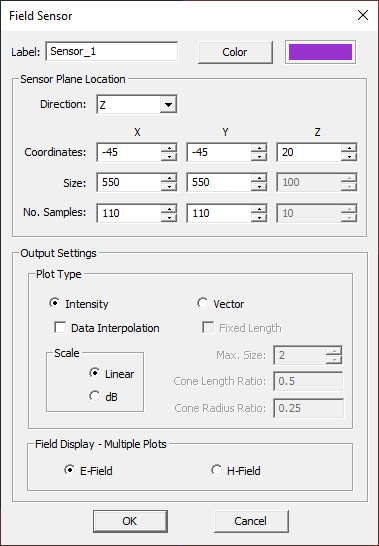EM.Illumina's near-field sensor dialog.

## Periodic Characteristics

MODULE: EM.Tempo, EM.Picasso

FUNCTION: Treats a periodic structure illuminated by a plane wave source as a periodic surface for computation of reflection and transmission coefficients

NOTE: If your physical structure has periodic domain settings along with a plane wave source, the reflection and transmission coefficients of the periodic surface are computed automatically at the end of the simulation. You don't need to explicitly define any observable for this purpose.

PERIODIC OUTPUT DATA FILES

Data File Name Data Type Description Notes & Restrictions
reflection_coefficient.CPX 2D complex scalar data real and imaginary parts of the reflection coefficient requires a plane wave source
transmission_coefficient.CPX 2D complex scalar data real and imaginary parts of the transmission coefficient requires a plane wave source

MODULE: EM.Terrano

FUNCTION: Defines a receiver set associated with an existing base location set

TO DEFINE A POINT RECEIVER SET:

1. Right-click on the Receivers item in the navigation tree of EM.Terrano.
2. Select Insert New Receiver Set... to open up the Receiver Set Dialog.
3. From the drop-down list labeled Select Base Point Set, choose the desired base location set, which can be a single point object or a point array.
5. You may also force the receivers to adjust their Z-coordinates based on the underlying terrain surface.
6. Click the OK button of the dialog to return to the project workspace. The new round symbols appear representing the receiver set.
7. You can open the property dialog of the receiver set and change the radiator type to User Defined Antenna. In that case, click the Import Pattern button of the dialog to set the file path for a far-field radiation pattern data file of ".RAD" type. You can also additionally rotate the imported radiation pattern about its local X-, Y- and Z-axes.

Parameter Name Value Type Units Default Value Notes
radiator type options: isotropic, user define antenna - isotropic -
pattern file file path - Models\DPL_STD.RAD imported far-field radiation pattern data file with a ".RAD" file extension for the case of user defined receiver antenna
rot_x real numeric degrees 0 additional rotation angle of the imported radiation pattern about the local X-axis
rot_y real numeric degrees 0 additional rotation angle of the imported radiation pattern about the local Y-axis
rot_z real numeric degrees 0 additional rotation angle of the imported radiation pattern about the local Z-axis

POINT RECEIVER SET'S OUTPUT DATA FILES

Data File Name Data Type Description
SBR_Results.RTOUT special output data file written by the SBR simulation engine individual ray data of each receiver
SBR_RecevierSetName_PATHLOSS.DAT 2D real scalar data the path loss between the transmitter and individual receivers of the receiver set as a function of receiver index
SBR_RecevierSetName_DELAY.DAT 2D real scalar data power of individual rays received by the selected receiver normalized by the power threshold
SBR_RecevierSetName_ThetaARRIVAL.DAT 2D real angular data the elevation angles of arrival of individual rays received by the selected receiver
SBR_RecevierSetName_PhiARRIVAL.DAT 2D real angular data the azimuth angles of arrival of individual rays received by the selected receiver
SBR_RecevierSetName_ThetaDEPARTURE.DAT 2D real angular data the elevation angles of departure of individual rays received by the selected receiver
SBR_RecevierSetName_PhiDEPARTURE.DAT 2D real angular data the azimuth angles of departure of individual rays received by the selected receiver
PREC_1.DAT 2D real scalar data the total received power by the selected receiver
PL_1.DAT 2D real | 2D real scalar data the path loss between the transmitter and the selected receiver
SBR_RecevierSetName.PRI special polarimetric ray information file represents the individual rays received by the selected receiver as plane wave sources

## Polar Graph

ICON: None

MODULE: EM.Tempo, EM.Illumina, EM.Picasso, EM.Libera

FUNCTION: Displays an angular data set where the independent and dependent variables are represented respectively by the angle measured counter-clockwise from the positive X-axis and radius

TO PLOT A POLAR GRAPH:

1. Select one or more data files with a .ANG file extension in the Data Manager and click the Plot button.
2. Alternatively, you can define two NumPy vectors ang and rad in the Python Interpreter and use the emag_plot_polarn(...) function.

PYTHON COMMAND(S): emag_plot_ang(file_name,title="",FRColor=(1,1,1),BKColor=(1,1,1),PLColor=(1,0,0),linewidth=1,linestyle="solid",marker="None",margins=(0.15,0.1,0.1,0.1,0.2,0.2,0.8,0.9,0.5,0.5),show_legend=True,*args,**kwargs)

emag_plot_two_ang(file_name1,file_name2,title="",FRColor=(1,1,1),BKColor=(1,1,1),PLColor1=(1,0,0),linewidth1=1,linestyle1="solid",marker1="None",PLColor2=(1,0,0),linewidth2=1,linestyle2="solid",marker2="None",margins=(0.15,0.1,0.1,0.1,0.2,0.2,0.8,0.9,0.5,0.5),show_legend=True,legend_label1="Default",legend_label2="Default",*args,**kwargs)

## Polar Stem Chart

ICON: None

MODULE: EM.Terrano

FUNCTION: Displays the radius values of an angular data set with a few discrete angle values

TO PLOT A POLAR STEM CHART:

1. The theta and phi angles of arrival and theta and phi angles of departure of the rays received by a specified receiver can be plotted on a polar stem chart.
2. Open EM.Terrano's Data Manager, select a delay profile data file with name similar to "SBR_ReceiverSet_1_PhiARRIVAL.ANG" or "SBR_ReceiverSet_1_ThetaDEPARTURE.ANG", and click the Plot button.

PYTHON COMMAND(S): None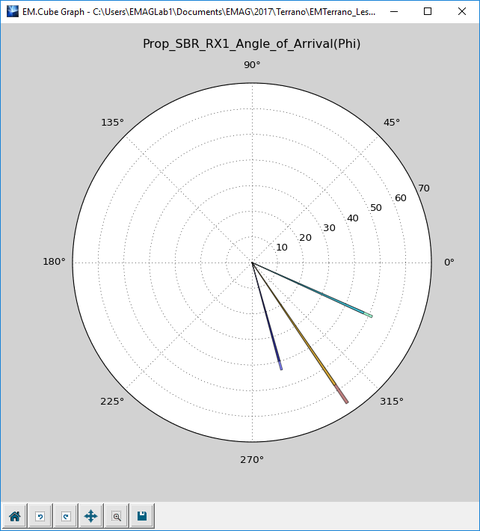A typical polar stem chart of a receiver's angles of arrival.

## Port Definition Observable

MODULE: EM.Tempo, EM.Picasso, EM.Libera

FUNCTION: Designates ports to the available sources for computation of S/Z/Y parameters

TO INITIATE A PORT DEFINITION:

1. Right-click on the Port Definitions item in the navigation tree.
2. Select Insert New Observable... to open up the Port Definition Dialog.
3. By default, one port is designated to each legitimate source.
4. By default, all the port have a reference impedance of 50 Ohms. You can change this value by editing the properties of each individual port.
5. Click the OK button of the dialog to return to the project workspace.

PYTHON COMMAND:

port_definition_default(label)

port_definition_custom(label,(port_1_src_1, port_1_src_2,...,port_1_impedance),(port_2_src_1, port_2_src_2,...,port_2_impedance),...)

PORT DEFINITION PARAMETERS

Parameter Name Value Type Units Default Value Notes
impedance real numeric Ohms 50 reference impedance for S-parameter computation
start frequency real numeric Hz fc-bw/2 lower end of frequency range for Fourier transform computation
end frequency real numeric Hz fc+bw/2 higher end of frequency range for Fourier transform computation

PORT OUTPUT DATA FILES

Data File Name Data Type Description Notes & Restrictions
Sij.CPX 2D complex scalar data real and imaginary parts of scattering parameter Sij EM.Picasso & EM.Libera
Zij.CPX 2D complex scalar data real and imaginary parts of impedance parameter Zij EM.Picasso & EM.Libera
Yij.CPX 2D complex scalar data real and imaginary parts of admittance parameter Yij EM.Picasso & EM.Libera
VSWR.DAT 2D real scalar data voltage standing wave ratio none
s_param_rfspice.TXT 2D real scalar data writes the S-parameters in Touchstone format for device generation in RF.Spice A/D none
DP_Sij.CPX 2D complex scalar data real and imaginary parts of scattering parameter Sij EM.Tempo
DP_Zij.CPX 2D complex scalar data real and imaginary parts of impedance parameter Zij EM.Tempo
DP_Yij.CPX 2D complex scalar data real and imaginary parts of admittance parameter Yij EM.Tempo
Current_Time_ij.DAT 2D real scalar data the current in port i due to the excitation of port j as a function of time EM.Tempo
Voltage_Time_ij.DAT 2D real scalar data the voltage across port i due to the excitation of port j as a function of time EM.Tempo
Current_Freq_ij.CPX 2D complex scalar data the real and imaginary parts of the current in port i due to the excitation of port j as a function of frequency EM.Tempo
Voltage_Freq_ij.CPX 2D complex scalar data the real and imaginary parts of the voltage across port i due to the excitation of port j as a function of frequency EM.Tempo
Power_Freq_ij.CPX 2D complex scalar data the real and imaginary parts of the power at port i due to the excitation of port j as a function of frequency EM.Tempo

## Radar Cross Section (RCS) Observable

MODULE: EM.Tempo, EM.Illumina, EM.Libera, EM.Picasso

FUNCTION: Computes the radar cross section in the spherical coordinate system

TO DEFINE AN RCS:

1. Right-click on the Radar Cross Sections item in the navigation tree.
2. Select Insert New RCS... to open up the RCS Dialog.
3. Change the theta and phi observation angle increments, if necessary. The default increment values are 5° for both angles.
4. By default, bistatic RCS is computed. You can choose monostatic RCS instead. In that case, a sweep simulation will be performed whereby the theta and phi incident angles of the plane wave source will vary at the same increments of the theta and phi observation angles.
5. In EM.Tempo, you can also choose the "Polarimetric Scattering Matrix Sweep" option. In this case, a sweep simulation will be performed whereby the theta and phi incident angles of the plane wave source will vary at the specified source theta and phi observation angles. Both TM and TE polarizations will be computed at each sweep.
6. Click the OK button of the dialog to return to the project workspace.

PYTHON COMMAND:

rcs_bistatic(label,theta_incr,phi_incr[,frequency])

rcs_monostatic(label,theta_incr,phi_incr[,frequency])

RCS PARAMETERS

Parameter Name Value Type Units Default Value Notes
RCS frequency real numeric Hz fc by default, the project's center frequency is assumed
observation phi angle increment real numeric degrees 5 resolution of computed radiation pattern along phi
observation theta angle increment real numeric degrees 5 resolution of computed radiation pattern along theta
non-principal phi plane real numeric degrees 45 additional plane cut
custom azimuth real numeric degrees 0 phi angle for computation of additional radiation characteristics
custom elevation real numeric degrees 0 theta angle for computation of additional radiation characteristics
source phi angle increment real numeric degrees 5 resolution of plane wave source's incident angle along phi when polarimetric scattering matrix sweep is selected
source theta angle increment real numeric degrees 5 resolution of plane wave source's incident angle along theta when polarimetric scattering matrix sweep is selected

RCS OUTPUT DATA FILES

Data File Name Data Type Description Notes & Restrictions
RCSName.RCS Spherical complex data frequency-domain σθ and σφ RCS data none
RCSName_RCS_Cart_XY.DAT 2D real scalar data frequency-domain 2D Cartesian RCS pattern in the XY plane none
RCSName_RCS_Cart_YZ.DAT 2D real scalar data frequency-domain 2D Cartesian RCS pattern in the YZ plane none
RCSName_RCS_Cart_ZX.DAT 2D real scalar data frequency-domain 2D Cartesian RCS pattern in the ZX plane none
RCSName_RCS_Cart_Custom.DAT 2D real scalar data frequency-domain 2D Cartesian RCS pattern in the custom phi-plane custom phi is 45° by default
RCSName_RCS_Polar_XY.ANG 2D real angular data frequency-domain 2D Polar RCS pattern in the XY plane none
RCSName_RCS_Polar_YZ.ANG 2D real angular data frequency-domain 2D Polar RCS pattern in the YZ plane none
RCSName_RCS_Polar_ZX.ANG 2D real angular data frequency-domain 2D Polar RCS pattern in the ZX plane none
RCSName_RCS_Polar_Custom.ANG 2D real angular data frequency-domain 2D Polar RCS pattern in the custom phi-plane custom phi is 45° by default
BRCS.DAT 2D real scalar data back-scatter RCS for the given plane wave incidence none
FRCS.DAT 2D real scalar data forward-scatter RCS for the given plane wave incidence none
MRCS.DAT 2D real scalar data maximum RCS for the given plane wave incidence none
MainBeam.DAT 2D real scalar data the elevation and azimuth angles of the main beam none
polar_scat.DAT 2D real scalar data polarimetric scattering matrix data for different incident angles of the plane wave source none

## Scatter Plot

ICON: None

FUNCTION: Displays the variation of a real-valued scalar data set with one, two or three independent variables.

TO PLOT A CARTESIAN GRAPH:

1. Select a data file with a .DAT file extension in the Data Manager and click the Graph Settings button.
2. In the Graph Settings dialog, select the Scatter option from the Graph Type drop-down list. Click the OK button to close the dialog and return to the Data Manager.
3. Click the Plot button of Data Manager to plot the scatter graph.

PYTHON COMMAND(S): emag_plot_dat(file_name,auto_scale=True,title="",xlabel="Default",ylabel="Default",FRColor=(1,1,1),BKColor=(1,1,1),PLColor=(1,0,0),linewidth=1,linestyle="solid",marker="None",margins=(0.15,0.1,0.1,0.1,0.2,0.2,0.8,0.9,0.5,0.5),show_major_grid=True,show_minor_grid=False,MAJColor=(0.25,0.25,0.25),MINColor=(0.5,0.5,0.5),major_grid_linewidth=1,minor_grid_linewidth=1,major_grid_linestyle="dotted",minor_grid_linestyle="dotted",axis_param=(0,0,5,5,0,0,5,5),show_legend=True,legend_label1="Default",legend_label2="Default",show_textbox=False,annot_text="",font_size=10,TXColor=(0,0,0),*args,**kwargs)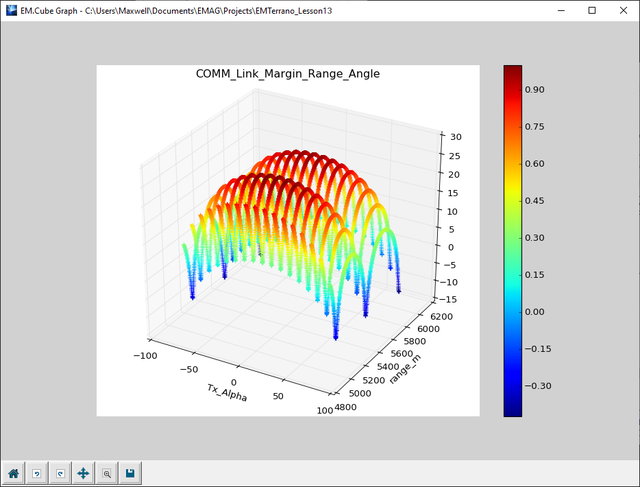A typical scatter plot of link margin vs. both range and transmit antenna’s rotation angle.

## Smith Chart

ICON: None

MODULE: EM.Tempo, EM.Picasso, EM.Libera

FUNCTION: Displays a complex-valued scalar data set on a Smith chart

TO PLOT A SMITH CHART:

1. First, select a data file with a .CPX file extension in the Data Manager.
2. Next, click the Graph Settings button to open the graph settings dialog. From the drop-down list labeled Graph Type, choose the Smith option.
3. Close the graph settings dialog and return to the Data Manager. With the complex data file selected, click the Plot button of Data Manager.
4. Alternatively, you can define a complex-valued NumbPy vector Z in the Python Interpreter and use the emag_plot_smith(...) function.

PYTHON COMMAND(S): emag_plot_cpx(file_name,auto_scale=True,title="",xlabel="Default",ylabel="Default",FRColor=(1,1,1),BKColor=(1,1,1),PLColor1=(1,0,0),linewidth1=1,linestyle1="solid",marker1="None",PLColor2=(1,0,0),linewidth2=1,linestyle2="solid",marker2="None",margins=(0.15,0.1,0.1,0.1,0.2,0.2,0.8,0.9,0.5,0.5),show_major_grid=True,show_minor_grid=False,MAJColor=(0.25,0.25,0.25),MINColor=(0.5,0.5,0.5),major_grid_linewidth=1,minor_grid_linewidth=1,major_grid_linestyle="dotted",minor_grid_linestyle="dotted",axis_param=(0,0,5,5,0,0,5,5),show_legend=True,legend_label1="Default",legend_label2="Default",show_textbox=False,annot_text="",font_size=10,TXColor=(0,0,0),*args,**kwargs)

emag_plot_smith(z_complex,title="New Graph",*args,**kwargs)

## Spherical Data Plot

ICON: None

FUNCTION: Displays the variation of a 3D spherical data set of the form r = f(θ,φ)

TO PLOT A CARTESIAN GRAPH:

1. You can visualize 3D far-field radiation pattern data or 3D radar cross section (RCS) data as spherical data plots.
2. Select a radiation pattern data file with a .RAD file extension or a 3D RCS data file with a .RCS file extension in the Data Manager's "3D Data Files" tab and click the Plot button of Data Manager.
3. The auto-scale features of the spherical data plots is enabled by default. In some cases, you may want to disable this feature by clicking the Graph Settings of Data Manager and unchecking the check box labeled Use Auto-Scale in the graph settings dialog.

emag_plot_rcs(file_name,auto_scale=True,title="",FRColor=(1,1,1),BKColor=(1,1,1),margins=(0.15,0.1,0.1,0.1,0.2,0.2,0.8,0.9,0.5,0.5),*args,**kwargs)A typical spherical data plot of 3D radiation pattern with the auto-scale feature enabled.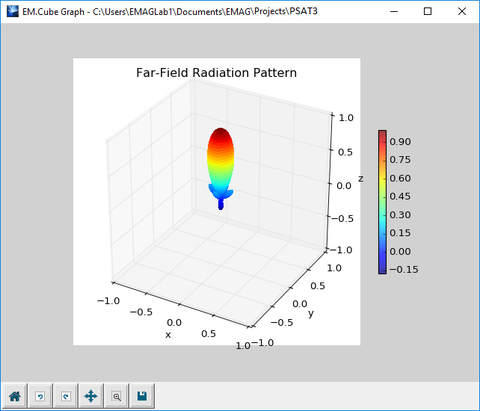A typical spherical data plot of 3D radiation pattern with the auto-scale feature disabled.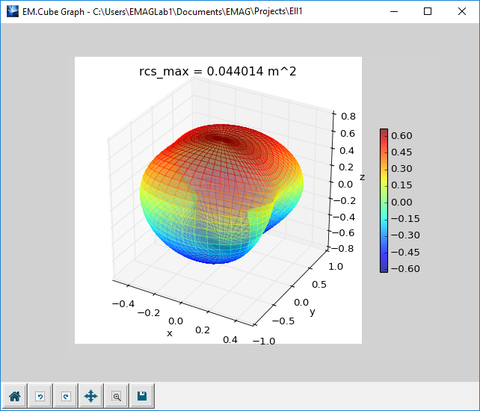A typical spherical data plot of 3D RCS with the auto-scale feature enabled.A typical spherical data plot of 3D RCS with the auto-scale feature disabled.

## Standard Output Data

At the end of an EM.Cube simulation, a number of computed quantities are designated as "Standard Output" parameters and can be used for various post-processing data operations. For example, you can define design objectives based on them, which you need for optimization.

The table below gives a list of all the currently available standard output parameters in EM.Cube along with the relevant computational modules:

Standard Output Name / Syntax Description Computational Modules
SijM Magnitude of (i,j)-th Scattering Parameter EM.Tempo, EM.Picasso, EM.Libera
SijP Phase of (i,j)-th Scattering Parameter (in radians) EM.Tempo, EM.Picasso, EM.Libera
SijR Real Part of (i,j)-th Scattering Parameter EM.Tempo, EM.Picasso, EM.Libera
SijI Imaginary Part of (i,j)-th Scattering Parameter EM.Tempo, EM.Picasso, EM.Libera
ZijM Magnitude of (i,j)-th Impedance Parameter EM.Tempo, EM.Picasso, EM.Libera
ZijP Phase of (i,j)-th Impedance Parameter (in radians) EM.Tempo, EM.Picasso, EM.Libera
ZijR Real Part of (i,j)-th Impedance Parameter EM.Tempo, EM.Picasso, EM.Libera
ZijI Imaginary Part of (i,j)-th Impedance Parameter EM.Tempo, EM.Picasso, EM.Libera
YijM Magnitude of (i,j)-th Admittance Parameter EM.Tempo, EM.Picasso, EM.Libera
YijR Real Part of (i,j)-th Admittance Parameter EM.Tempo, EM.Picasso, EM.Libera
YijI Imaginary Part of (i,j)-th Admittance Parameter EM.Tempo, EM.Picasso, EM.Libera
VSWR Voltage Standing Wave Ratio EM.Tempo, EM.Picasso, EM.Libera
D0 Directivity EM.Tempo, EM.Picasso, EM.Libera, EM.Illumina, EM.Terrano
REFF Radiation Efficiency EM.Tempo, EM.Picasso, EM.Libera
G0 Antenna Gain EM.Tempo, EM.Picasso, EM.Libera
THM Main Beam Theta EM.Tempo, EM.Picasso, EM.Libera, EM.Illumina, EM.Terrano
PHM Main Beam Phi EM.Tempo, EM.Picasso, EM.Libera, EM.Illumina, EM.Terrano
DGU Directive Gain along User Defined Direction EM.Tempo, EM.Picasso, EM.Libera, EM.Illumina, EM.Terrano
ARU Axial Ratio along User Defined Direction EM.Tempo, EM.Picasso, EM.Libera, EM.Illumina, EM.Terrano
FBR Front-to-Back Ratio EM.Tempo, EM.Picasso, EM.Libera, EM.Illumina, EM.Terrano
HPBWXY Half Power Beam Width in XY Plane EM.Tempo, EM.Picasso, EM.Libera, EM.Illumina, EM.Terrano
HPBWYZ Half Power Beam Width in YZ Plane EM.Tempo, EM.Picasso, EM.Libera, EM.Illumina, EM.Terrano
HPBWZX Half Power Beam Width in ZX Plane EM.Tempo, EM.Picasso, EM.Libera, EM.Illumina, EM.Terrano
HPBWU Half Power Beam Width in User Defined Plane EM.Tempo, EM.Picasso, EM.Libera, EM.Illumina, EM.Terrano
SLLXY Maximum Side Lobe Level in XY Plane EM.Tempo, EM.Picasso, EM.Libera, EM.Illumina, EM.Terrano
SLLYZ Maximum Side Lobe Level in YZ Plane EM.Tempo, EM.Picasso, EM.Libera, EM.Illumina, EM.Terrano
SLLZX Maximum Side Lobe Level in ZX Plane EM.Tempo, EM.Picasso, EM.Libera, EM.Illumina, EM.Terrano
SLLU Maximum Side Lobe Level in User Defined Plane EM.Tempo, EM.Picasso, EM.Libera, EM.Illumina, EM.Terrano
FNBXY First Null Beam Width in XY Plane EM.Tempo, EM.Picasso, EM.Libera, EM.Illumina, EM.Terrano
FNBYZ First Null Beam Width in YZ Plane EM.Tempo, EM.Picasso, EM.Libera, EM.Illumina, EM.Terrano
FNBZX First Null Beam Width in ZX Plane EM.Tempo, EM.Picasso, EM.Libera, EM.Illumina, EM.Terrano
FNBU First Null Beam Width in User Defined Plane EM.Tempo, EM.Picasso, EM.Libera, EM.Illumina, EM.Terrano
FNLXY First Null Level in XY Plane EM.Tempo, EM.Picasso, EM.Libera, EM.Illumina, EM.Terrano
FNLYZ First Null Level in YZ Plane EM.Tempo, EM.Picasso, EM.Libera, EM.Illumina, EM.Terrano
FNLZX First Null Level in ZX Plane EM.Tempo, EM.Picasso, EM.Libera, EM.Illumina, EM.Terrano
FNLU First Null Level in User Defined Plane EM.Tempo, EM.Picasso, EM.Libera, EM.Illumina, EM.Terrano
BRCS Back-Scatter RCS EM.Tempo, EM.Picasso, EM.Libera, EM.Illumina
FRCS Forward-Scatter RCS along User Defined Incident Direction EM.Tempo, EM.Picasso, EM.Libera, EM.Illumina
MRCS Maximum Bi-static RCS EM.Tempo, EM.Picasso, EM.Libera, EM.Illumina
RCM Magnitude of Reflection Coefficient EM.Tempo, EM.Picasso
RCP Phase of Reflection Coefficient (in radians) EM.Tempo, EM.Picasso
RCR Real Part of Reflection Coefficient EM.Tempo, EM.Picasso
RCI Imaginary Part of Reflection Coefficient EM.Tempo, EM.Picasso
TCM Magnitude of Transmission Coefficient EM.Tempo, EM.Picasso
TCP Phase of Transmission Coefficient (in radians) EM.Tempo, EM.Picasso
TCR Real Part of Transmission Coefficient EM.Tempo, EM.Picasso
TCI Imaginary Part of Transmission Coefficient EM.Tempo, EM.Picasso
SNR_i Signal-to-Noise Ratio Path at the User-Specified Receiver in Receiver Set i EM.Terrano
PL_i Path Loss from the Transmitter to the User-Specified Receiver in Receiver Set i EM.Terrano
name_Z0 Characteristic Impedance of a 2D Transmission Line defined by the Solution Plane "name" EM.Ferma
name_EEFF Effective Permittivity of a 2D Transmission Line defined by the Solution Plane "name" EM.Ferma
name_voltage Voltage Calculated by the Field Integral Observable "name" EM.Ferma
name_current Loop Current Calculated by the Field Integral Observable "name" EM.Ferma
name_EFlux Electric Flux Calculated by the Field Integral Observable "name" EM.Ferma
name_HFlux Magnetic Flux Calculated by the Field Integral Observable "name" EM.Ferma
name_EEnergy Electric Energy Calculated by the Field Integral Observable "name" EM.Ferma
name_HEnergy Magnetic Energy Calculated by the Field Integral Observable "name" EM.Ferma
name_conduction Conduction Current Calculated by the Field Integral Observable "name" EM.Ferma
name_ohmic Ohmic Power Loss Calculated by the Field Integral Observable "name" EM.Ferma
name_Cap Capacitance Calculated by the Field Integral Observable "name" EM.Ferma
name_Ind Inductance Calculated by the Field Integral Observable "name" EM.Ferma
name_Res Resistance Calculated by the Field Integral Observable "name" EM.Ferma

All the port characteristics involving S/Z/Y parameters are available only if your project has a port definition. SijM, etc. means the scattering parameter observed at port i due to a source excited at port j. Similar definitions apply to all the S, Z and Y parameters. If your structure has N ports, there will be a total of N2 scattering parameters, a total of N2 impedance parameters, and a total of N2 admittance parameters. Additionally, there are four standard output parameters associated with each of the individual S/Z/Y parameters: magnitude, phase (in radians), real part and imaginary part. The same is true for the reflection and transmission coefficients of a periodic structure. Each coefficient has four associated standard output parameters. The reflection and transmission coefficient parameters, of course, are available only if your structure has a periodic domain and is excited by a plane wave source.

All the radiation- and scattering-related standard outputs are available only if you have defined a radiation pattern far field observable or an RCS far field observable, respectively. The standard output parameters DGU and ARU are the directive gain and axial ratio calculated at the user-specified direction with spherical observation angles (θ, φ). These angles are specified in degrees as Custom Azimuth & Elevation Angles in the "Output Settings" section of the Radiation Pattern Dialog. The standard output parameters HPBWU, SLLU, FNBU and FNLU are determined at a user defined phi-plane cut. This azimuth angle is specified in degrees as Non-Principal Phi Plane in the "Output Settings" section of the Radiation Pattern Dialog, and its default value is 45°. The standard output parameters BRCS and MRCS are the total back-scatter RCS and the maximum total RCS of your target structure when it is excited by an incident plane wave source at the specified θs and φs source angles. FRCS, on the other hand, is the total forward-scatter RCS measured at the predetermined θo and φo observation angles. These angles are specified in degrees as Custom Azimuth & Elevation Angles in the "Output Settings" section of the Radar Cross Section Dialog.

## Static Field Integral Observable

MODULE: EM.Ferma

FUNCTION: Computes a number of different static quantities involving electric and magnetic field integrals

TO DEFINE A FIELD INTEGRAL:

1. Right-click on the Static Field Integrals item in the navigation tree of EM.Ferma.
2. Select Insert New Observable... to open up the Field Integral Dialog.
3. From Field Integral Type drop-down list, choose one of the eleven type options.
4. Most options involve a single field integral. Capacitance, Self-Inductance, Mutual Inductance and Resistance involve two field integrals.
5. Each field integral might be a line, closed loop, open surface, closed surface or volume integral depending the selected type option. The domain of all of these integrals are defined by two opposite corner points. You need to enter the coordinated of these two opposite corners.
6. Click the OK button of the dialog to return to the project workspace.

NOTES, SPECIAL CASES OR EXCEPTIONS: Box domains are specified by the coordinates of two opposite corners. Voltage Path requires a line; therefore, two of the coordinates of the two corners must be identical. Otherwise, an error message will pop up. For example, (0, 0, 0) for Corner 1 and (10, 0, 0) for Corner 2 define a Z-directed line segment. Current Loop requires a rectangle; therefore, one of the coordinates of the two corners must be identical. For example, (0, 0, 0) for Corner 1 and (10, 10, 0) for Corner 2 define a rectangle in the XY plane.

PYTHON COMMAND:

voltage_integral(label,x1,y1,z1,x2,y2,z2)

current_integral(label,x1,y1,z1,x2,y2,z2)

conduction_current_integral(label,x1,y1,z1,x2,y2,z2)

flux_electric(label,x1,y1,z1,x2,y2,z2)

flux_magnetic(label,x1,y1,z1,x2,y2,z2)

energy_electric(label,x1,y1,z1,x2,y2,z2)

energy_magnetic(label,x1,y1,z1,x2,y2,z2)

ohmic_loss(label,x1,y1,z1,x2,y2,z2)

capacitance(label,x1,y1,z1,x2,y2,z2,x3,y3,z3,x4,y4,z4)

capacitance_energy(label,x1,y1,z1,x2,y2,z2,x3,y3,z3,x4,y4,z4)

inductance(label,x1,y1,z1,x2,y2,z2,x3,y3,z3,x4,y4,z4)

inductance_energy(label,x1,y1,z1,x2,y2,z2,x3,y3,z3,x4,y4,z4)

inductance_mutual(label,x1,y1,z1,x2,y2,z2,x3,y3,z3,x4,y4,z4)

resistance(label,x1,y1,z1,x2,y2,z2,x3,y3,z3,x4,y4,z4)

resistance_power_voltage(label,x1,y1,z1,x2,y2,z2,x3,y3,z3,x4,y4,z4)

resistance_power_current(label,x1,y1,z1,x2,y2,z2,x3,y3,z3,x4,y4,z4)

flux_thermal(label,x1,y1,z1,x2,y2,z2)

energy_thermal(label,x1,y1,z1,x2,y2,z2)

FIELD INTEGRAL PARAMETERS

Parameter Name Value Type Units Default Value Notes
field integral type option: voltage, current, conduction current, electric flux, magnetic flux, electric energy, magnetic energy, ohmic power loss, capacitance, inductance, resistance - voltage -
x1 real numeric project units -10.0 X-coordinate of Corner 1 of the first field integral
y1 real numeric project units -10.0 Y-coordinate of Corner 1 of the first field integral
z1 real numeric project units -10.0 Z-coordinate of Corner 1 of the first field integral
x2 real numeric project units 10.0 X-coordinate of Corner 2 of the first field integral
y2 real numeric project units 10.0 Y-coordinate of Corner 2 of the first field integral
z2 real numeric project units 10.0 Z-coordinate of Corner 2 of the first field integral
x3 real numeric project units 0 X-coordinate of Corner 1 of the second field integral
y3 real numeric project units 0 Y-coordinate of Corner 1 of the second field integral
z3 real numeric project units 0 Z-coordinate of Corner 1 of the second field integral
x4 real numeric project units 0 X-coordinate of Corner 2 of the second field integral
y4 real numeric project units 0 Y-coordinate of Corner 2 of the second field integral
z4 real numeric project units 0 Z-coordinate of Corner 2 of the second field integral

FIELD INTEGRAL DEFINITIONS

Field Integral Definition Notes
Voltage $V = - \int_C \mathbf{E(r)} . \mathbf{dl}$ C represents an open curve (path)
Current $I = \oint_{C_o} \mathbf{H(r)} . \mathbf{dl}$ Co represents a closed curve (loop)
Conduction Current $I_{cond} = \int\int_S \mathbf{J(r)} . \mathbf{ds} = \int\int_S \sigma \mathbf{E(r)} . \mathbf{ds}$ S represents an open surface like a plane
Electric Flux $\Phi_E = \int\int_{S_o} \mathbf{D(r)} . \mathbf{ds} = \int\int_{S_o} \epsilon \mathbf{E(r)} . \mathbf{ds}$ So represents a closed surface like a box
Magnetic Flux $\Phi_H = \int\int_S \mathbf{B(r)} . \mathbf{ds} = \int\int_S \mu \mathbf{H(r)} . \mathbf{ds}$ S represents an open surface like a plane
Electric Energy $W_E = \frac{1}{2} \int \int \int_V \epsilon \vert \mathbf{E(r)} \vert ^2 dv$ V represents a volume like a box
Magnetic Energy $W_H = \frac{1}{2} \int\int\int_V \mu \vert \mathbf{H(r)} \vert ^2 dv$ V represents a volume like a box
Ohmic Power Loss $P_{ohmic} = \int\int\int_V \sigma \vert \mathbf{E(r)} \vert ^2 dv$ V represents a volume like a box
Capacitance $C = \Phi_E/V = \int\int_{S_o} \epsilon \mathbf{E(r)} . \mathbf{ds} / \int_C \mathbf{E(r)} . \mathbf{dl}$ Ratio of two field integrals
Capacitance (Alternative) $C = 2W_E/V^2 = 2 \int \int \int_V \epsilon \vert \mathbf{E(r)} \vert ^2 dv / \left( \int_C \mathbf{E(r)} . \mathbf{dl} \right)^2$ Energy-based definition
Self-Inductance $L = \Phi_H/I = \int\int_S \mu \mathbf{H(r)} . \mathbf{ds} / \oint_{C_o} \mathbf{H(r)} . \mathbf{dl}$ Ratio of two field integrals
Self-Inductance (Alternative) $L = 2W_M/I^2 = 2 \int \int \int_V \mu \vert \mathbf{H(r)} \vert ^2 dv / \left( \oint_{C_o} \mathbf{H(r)} . \mathbf{dl} \right)^2$ Energy-based definition
Mutual Inductance $M = \Phi_H^{\prime}/I = \int\int_{S^{\prime}} \mu \mathbf{H(r)} . \mathbf{ds} / \oint_{C_o} \mathbf{H(r)} . \mathbf{dl}$ S' represents an open surface or plane passing through the second (coupled) inductor and Φ'H represents the magnetic flux linkage due to the magnetic field of the first inductor passing through the second inductor
Resistance $R = V/I_{cond} = - \int_C \mathbf{E(r)} . \mathbf{dl} / \int\int_S \sigma \mathbf{E(r)} . \mathbf{ds}$ Ratio of two field integrals
Resistance (Alternative 1) $R = V^2/P_{ohmic} = \left( \int_C \mathbf{E(r)} . \mathbf{dl} \right)^2 / \int\int\int_V \sigma \vert \mathbf{E(r)} \vert ^2 dv$ Power-based definition
Resistance (Alternative 2) $R = P_{ohmic}/I_{cond}^2 = \int\int\int_V \sigma \vert \mathbf{E(r)} \vert ^2 dv / \left( \int\int_S \sigma \mathbf{E(r)} . \mathbf{ds} \right)^2$ Power-based definition
Thermal Flux $\Phi_T = \int\int_{S_o} \mathbf{q(r)} . \mathbf{ds}$ So represents a closed surface like a box
Thermal Energy $W_T = Q = \int \int \int_V \rho_V c_p \left( T\mathbf{(r)} - T_{env} \right) dv$ V represents a volume like a box

FIELD INTEGRAL OUTPUT DATA FILES

Data File Name Data Type Description
Voltage.DAT 2D real scalar data voltage computed from the integral of E-field
Current.DAT 2D real scalar data current computed from Ampere's loop integral of H-field
Conduction_Current.DAT 2D real scalar data total current computed from the surface integral of volume current density J = σE
flux_E.DAT 2D real scalar data the total electric flux passing through a specified box
flux_H.DAT 2D real scalar data the total magnetic flux passing through a specified rectangular plane
energy_E.DAT 2D real scalar data the total electric energy stored in a specified box
energy_H.DAT 2D real scalar data the total magnetic energy stored in a specified box
ohmic.DAT 2D real scalar data the total Ohmic power dissipated in a specified box
capacitance.DAT 2D real scalar data capacitance computed from the electric flux and voltage
inductance.DAT 2D real scalar data self-inductance computed from the magnetic flux and current
mutual_inductance.DAT 2D real scalar data mutual inductance between two objects computed from the magnetic flux and current
resistance.DAT 2D real scalar data resistance computed from the voltage and conduction current
flux_T.DAT 2D real scalar data the total thermal flux passing through a specified box
energy_T.DAT 2D real scalar data the total thermal energy stored in a specified box

## Surface Plot

ICON: None

FUNCTION: Displays the variation of a 3D Cartesian data set of the form z = f(x,y) on a specified principal plane

TO PLOT A CARTESIAN GRAPH:

1. You can visualize 3D Cartesian data or electric and magnetic field distributions as 3D surface plots.
2. Select a 3D Cartesian data file iwth a .CAR file extension or a field sensor data file with a .SEN file extension in the Data Manager's "3D Data Files" tab and click the Graph Settings button.
3. From the drop-down list labeled Graph Type, choose the Surface option. Close the graph settings dialog and return to the Data Manager. With the 3D data file selected, click the Plot button of Data Manager.
4. Alternatively, you can define two NumPy vectors X and Y and a matrix Z in the Python Interpreter and use the emag_plot_surface(...) function.

PYTHON COMMAND(S): emag_plot_surface(x,y,z_matrix,title="New Graph",x_label="x",y_label="y",*args,**kwargs)

emag_plot_car_surf(file_name,title="",FRColor=(1,1,1),BKColor=(1,1,1),margins=(0.15,0.1,0.1,0.1,0.2,0.2,0.8,0.9,0.5,0.5),*args,**kwargs)

emag_plot_sen_surf(file_name,title="",FRColor=(1,1,1),BKColor=(1,1,1),margins=(0.15,0.1,0.1,0.1,0.2,0.2,0.8,0.9,0.5,0.5),*args,**kwargs)

emag_plot_sen_tempo_surf(file_name,input_name,grid_name,title="",FRColor=(1,1,1),BKColor=(1,1,1),margins=(0.15,0.1,0.1,0.1,0.2,0.2,0.8,0.9,0.5,0.5),*args,**kwargs)

emag_plot_sen_ferma_surf(file_name,title="",FRColor=(1,1,1),BKColor=(1,1,1),margins=(0.15,0.1,0.1,0.1,0.2,0.2,0.8,0.9,0.5,0.5),*args,**kwargs)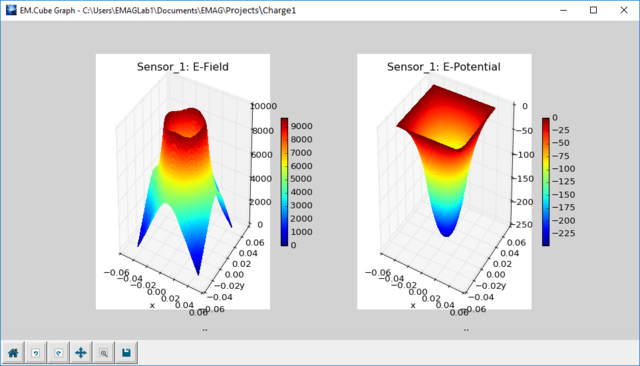A typical surface plot of electric field and potential.

## Temporal Field Probe Observable

MODULE: EM.Tempo

FUNCTION: Monitors time-domain and frequency-domain electric and magnetic field components at a specified point in space

TO DEFINE A FIELD PROBE:

1. Right-click on the Temporal Field Probes item in the navigation tree of EM.Tempo.
2. Select Insert New Observable... to open up the Field Probe Dialog.
3. By default, the field probe is placed at the origin of coordinates. Enter new (X,Y,Z) coordinates for the desired location.
4. Click the OK button of the dialog to return to the project workspace.

NOTES, SPECIAL CASES OR EXCEPTIONS: By default, all the six electric and magnetic field components are computed at the center of the Yee cell. This involves interpolation and averaging among neighboring cells. If you uncheck the box labeled "Compute Fields at the Center of Yee Cell", then the probe field components will reflect those of the original Yee cell locations.

PYTHON COMMAND: field_probe(label,x0,y0,z0)

FIELD PROBE PARAMETERS

Parameter Name Value Type Units Default Value Notes
x0 real numeric project units 0 X-coordinates of probe location
y0 real numeric project units 0 Y-coordinate of probe location
z0 real numeric project units 0 Z-coordinate of probe location
start frequency real numeric Hz fc-bw/2 lower end of frequency range for Fourier transform computation
end frequency real numeric Hz fc+bw/2 higher end of frequency range for Fourier transform computation

FIELD PROBE'S OUTPUT DATA FILES

Data File Name Data Type Description
ProbeName_X_E_Time.DAT 2D real scalar data time-domain Ex electric field data
ProbeName_Y_E_Time.DAT 2D real scalar data time-domain Ey electric field data
ProbeName_Z_E_Time.DAT 2D real scalar data time-domain Ez electric field data
ProbeName_X_H_Time.DAT 2D real scalar data time-domain Hx magnetic field data
ProbeName_Y_H_Time.DAT 2D real scalar data time-domain Hy magnetic field data
ProbeName_Z_H_Time.DAT 2D real scalar data time-domain Hz magnetic field data
ProbeName_X_E_Fre.CPX 2D complex scalar data frequency-domain Ex electric field data
ProbeName_Y_E_Fre.CPX 2D complex scalar data frequency-domain Ey electric field data
ProbeName_Z_E_Fre.CPX 2D complex scalar data frequency-domain Ez electric field data
ProbeName_X_H_Fre.CPX 2D complex scalar data frequency-domain Hx magnetic field data
ProbeName_Y_H_Fre.CPX 2D complex scalar data frequency-domain Hy magnetic field data
ProbeName_Z_H_Fre.CPX 2D complex scalar data frequency-domain Hz magnetic field data

## u-v Plot

ICON: None

FUNCTION: Displays a far-field radiation pattern or RCS data file as a polar colorgrid plot in the u-v coordinate system.

TO PLOT A CARTESIAN GRAPH:

1. Select a data file with a .RAD or .RCS file extension in the Data Manager and click the Graph Settings button.
2. In the Graph Settings dialog, select the u-v option from the Graph Type drop-down list. Click the OK button to close the dialog and return to the Data Manager.
3. Click the Plot button of Data Manager to plot the vector-quiver graph

emag_plot_rcs_uv(file_name,db_mode=0,auto_scale=True,title="",FRColor=(1,1,1),BKColor=(1,1,1),margins=(0.15,0.1,0.1,0.1,0.2,0.2,0.8,0.9,0.5,0.5),db_ceil=0,db_floor=-50,nres=500,*args,**kwargs)

## u-v Surface Plot

ICON: None

FUNCTION: Displays a far-field radiation pattern or RCS data file as a surface plot in the u-v coordinate system.

TO PLOT A CARTESIAN GRAPH:

1. Select a data file with a .RAD or .RCS file extension in the Data Manager and click the Graph Settings button.
2. In the Graph Settings dialog, select the Surface u-v option from the Graph Type drop-down list. Click the OK button to close the dialog and return to the Data Manager.
3. Click the Plot button of Data Manager to plot the u-v surface graph

emag_plot_rcs_uv_surf(file_name,db_mode=0,auto_scale=True,title="",FRColor=(1,1,1),BKColor=(1,1,1),margins=(0.15,0.1,0.1,0.1,0.2,0.2,0.8,0.9,0.5,0.5),db_ceil=0,db_floor=-50,nres=200,*args,**kwargs)

## Vector-Quiver Plot

ICON: None

FUNCTION: Displays the variation of a real-valued or complex-valued vector data set using directed arrows aligned with the vector field.

TO PLOT A CARTESIAN GRAPH:

1. Select a data file with a .CAR file extension in the Data Manager and click the Graph Settings button.
2. In the Graph Settings dialog, select the Vector-Quiver option from the Graph Type drop-down list. Click the OK button to close the dialog and return to the Data Manager.
3. Click the Plot button of Data Manager to plot the vector-quiver graph
4. Note the Cartesian data files generated by EM.Tempo have (i,j,k) index coordinates and cannot be plotted as vector-quiver plots. To do so, you should first convert those Cartesian files to regular ones having (x,y,z) coordinates. This can be using Data Manager’s Convert utility

PYTHON COMMAND(S): emag_plot_car_vector(file_name,auto_scale=True,title="",FRColor=(1,1,1),BKColor=(1,1,1),PLColor=(1,0,0),margins=(0.15,0.1,0.1,0.1,0.2,0.2,0.8,0.9,0.5,0.5),length_ratio=0.3,arrow_ratio=0.5,is_stream=False,*args,**kwargs)